Properties of solutions to potential equations

## Linear Methods of Applied Mathematics Evans M. Harrell II and James V. Herod

version of 30 August 2000.

## Properties of potential equations

Even if there is an explicit formula for the solution of an equation, you may not have enough information about the boundary or intiial conditions to calculate that solution. Also, in its complexity, an explicit solution may hide important physical properties. For these among other reasons it is often useful analyze the features which will be shared by all solutions to a particular partial differential equation. If there are some such general features, we can hope to find them either by analyzing the equation itself or by analyzing a formula for the solution. For instance, the Green function for a partial differential equation must contain all the physical properties shared by the solutions it is capable of representing.

We begin by discussing the potential equations of Laplace and Poisson, which are discussed in Chapters VI, IX, and XIX. Our main tool will be integration by parts, as encapsulated by Green's identities.

The question we ask is the relationship between a function u(x) and its average value over a small sphere centered at x. The average would be expected to depend on the radius of the ball, which we'll call r. Thus: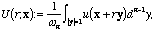wheren is the area of a sphere in n dimensions, of radius 1. Differentiating with the vector chain rule, we get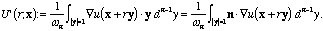This is now in a form where we can apply Green's first identity with the simplification that the function f is 1 (while g is u(x+ry)). The result is that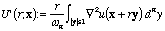(pr1)
(the factor r arises because of the chain rule: the gradient in Green's identity is with respect to y rather than x).

This formula has several interesting consequences:

Definition PR.1. A function is harmonic if it satisfies Laplace's equation,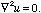.
It is subharmonic if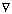2u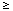0.
Finally, it is superharmonic if2u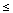0.
In these definitions, we assume that u is a smooth function and satisfies the differential equation or inequality on an open region. Note that any superharmonic function is the negative of a subharmonic function and vice versa, so they are not really distinct notions. Some sources define subharmonic and superharmonic functions by the property mentioned in Theorem PR2, below, rather than by a differential inequality.

From (pr1) we get the following theorem:

Theorem PR.2.

1. Suppose that u is harmonic on an open region D and that r is less than the distance from x to the boundary of D Then u = U(r;x). Moreover, u is also equal to its average over the ball {y: |y-x| < r}.
2. Suppose that u is subharmonic (resp. superharmonic) on an open region D as in part 1, Then u < U(r;x) (resp. u > U(r;x)). Moreover, in this statement the average over the sphere may be replaced by the average over the ball {y: |y-x| < r}.
3. If the derivatives of second order of a function u are continuous, the mean-value properties stated in parts 1 and 2 imply that u is harmonic, subharmonic, or superharmonic, accordingly.

Remarks about the proof. Replacing the spheres by the balls in parts 1 and 2 is merely a matter of performing an additional integration over r. Other than that these statements are immediate from (pr1). The converse, part 3, also follows from that formula, with contrapositive logic: We consider the converse of part 1, as representative. Suppose that2u is continuous and not equal to 0 at some point x. Then either there is some ball centered at x where the right side of (pr1) is positive, or else a ball centered at x where the right side of (pr1) is negative. Whichever statement applies, U(r,x) changes as r varies, which contradicts the mean-value property.

As an example of the physical consequences of this, consider heat flow, as in Chapter VIII. If the temperature distribution of a homogeneous body does not have the mean-value property guaranteed by Theorem PR.2 for harmonic functions, then it is not in equilibrium, and the temperature will redistribute itself.

The mean-value property is also related to the maximum principle, which was stated and proved for the heat equation in Chapter VIII. In the case of harmonic functions it reads as follows.

Theorem PR.3. Suppose that u is harmonic on an open region D. If u attains its maximum or minimum value within D then u must be a constant throughout D.

Another stetment of the maximum principle is that: for any closed region on which u is harmonic, its maximum and its minimum are attained on the boundary.

Finally, the maximum principle is an important tool for knowing when a problem is well-posed, for it implies a uniqueness theorem for Poisson's equation (see Chapter 19):

Theorem PR.4. Suppose that2u = f on D,
u = g on the boundary of D.
has two solutions, u1 and u2. Then u1 = u2.
```
```
Proof. Let w(x) := u1(x) - u2(x). Then we know that w solves Laplace's equation and is identically 0 on the boundary of D. As a consequence of the maximum principle, both the maximum and the minimum of w in D must equal 0, which means that u1 = u2.
```                                                                                          QED
```

Exercises PR
1. Provide two proofs for the maximum principle for harmonic functions:
2. State and prove maximum or minimum principles for subharmonic and superharmonic functions.